When you use workbooks, the proportion of data under certain conditions need to be calculated. To solve this problem, create a new field. Proportion problems are classified into two types. The first type is the proportion of similar orders. For example, you need to calculate the proportion occupied by the order amount of each product type in "North China" to the total amount in all areas. The other one is the proportion of the number of orders. For example, to calculate the proportion of the number of senior orders, you must calculate the number of senior orders and that of total orders.

The following example uses company_sales_record as its dataset.

## Prepare the dataset

Create a dataset based on the table company_sales_record and change the geographical dimension of the area parameter to: area.

## The new field shows the order amount in “North China”

On the dataset editing page, create a measure: order_amount_in_NorthChina, as shown in the following figure.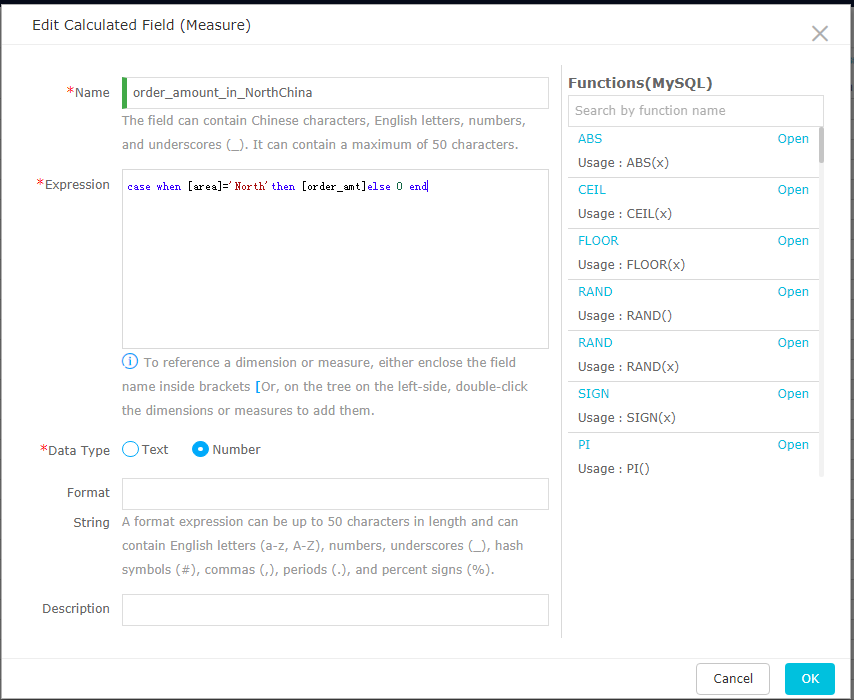## Create a field to calculate the proportion of the order amount in North China

On the dataset editing page, create a measure: proportion_order_amt_NorthChina, as shown in the following figure.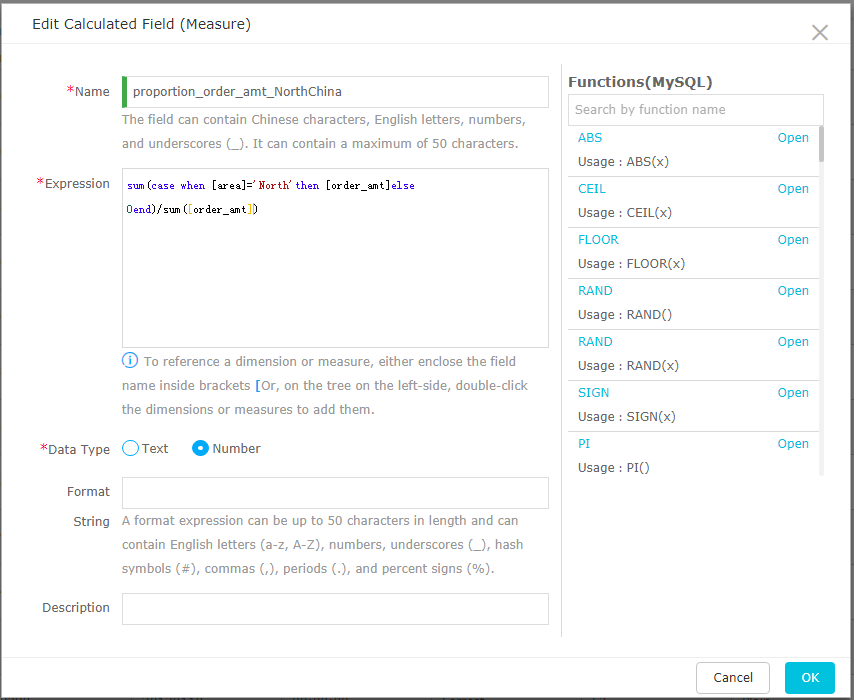## Save and refresh the dataset, and then create a new workbook

Save the dataset and create a new workbook. Select product_sub_type, order_amount_in_NorthChina, order_amt, and proportion of order amount in NorthChina, as shown in the following figure.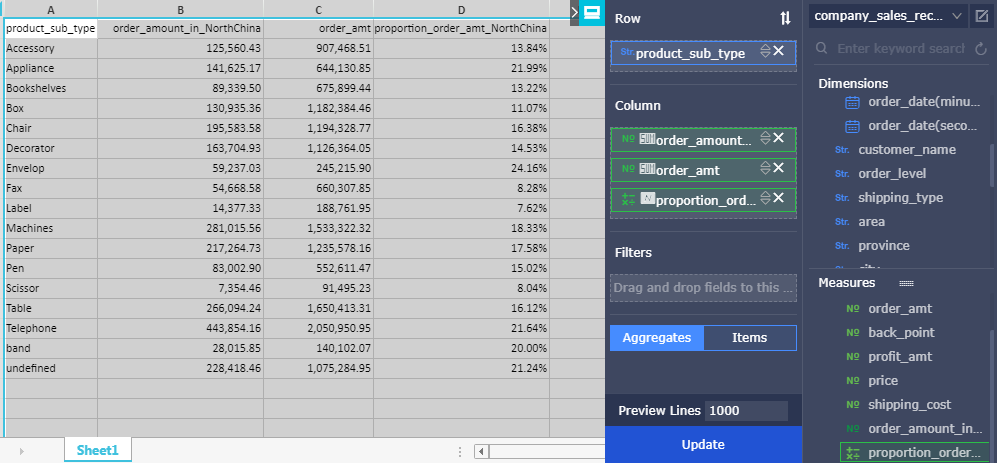## Save the workbook

Save the workbook. The amount proportion problem is solved.

## Create a new field to calculate the proportion of the number of senior orders

Create a measure to display the proportion of the number of senior orders.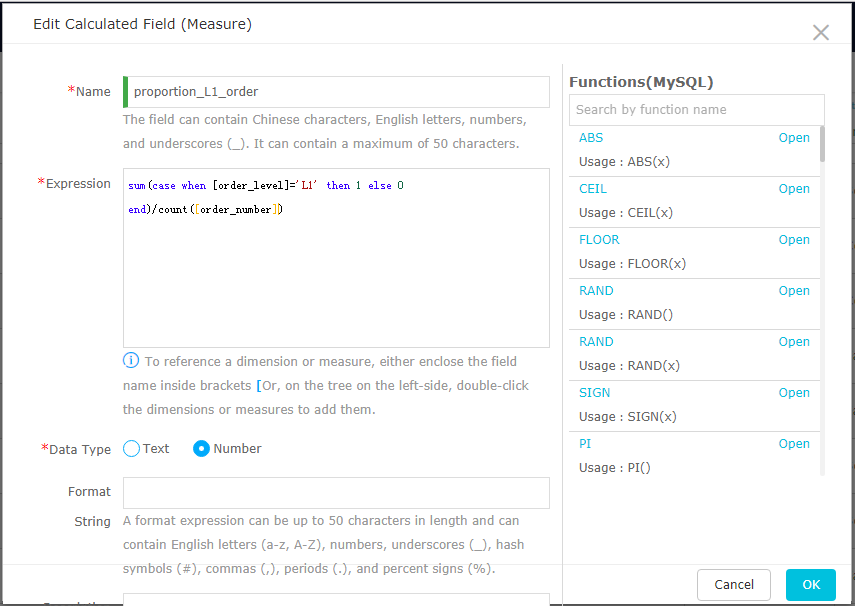## Save the dataset, and create a new workbook

Select area, proportion_L1_order, and order_number, as shown in the following figure.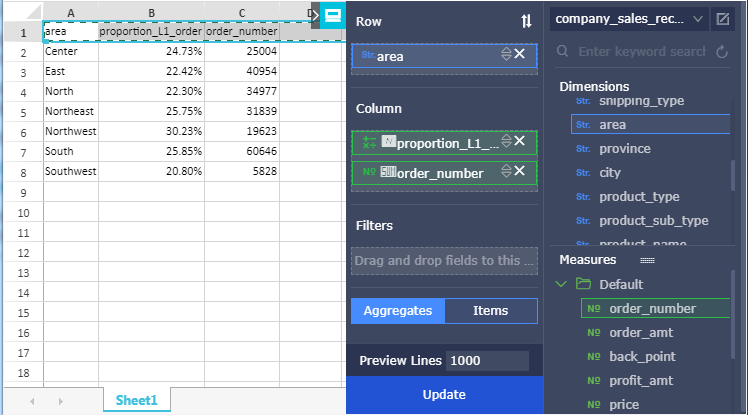## Save the workbook

Save the workbook. The number proportion problem is solved.